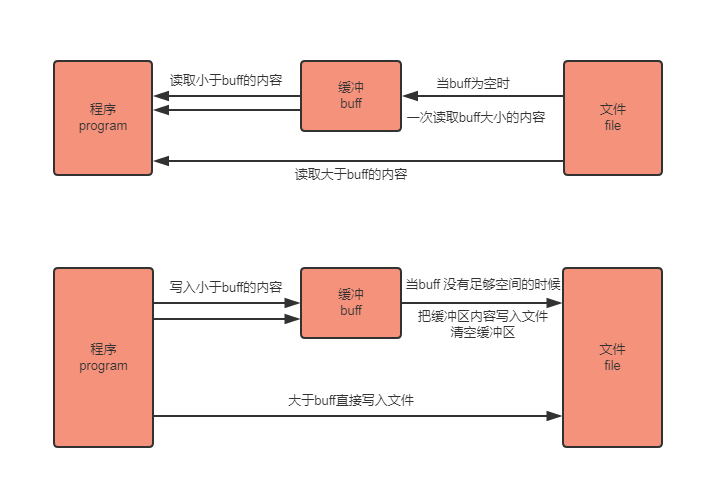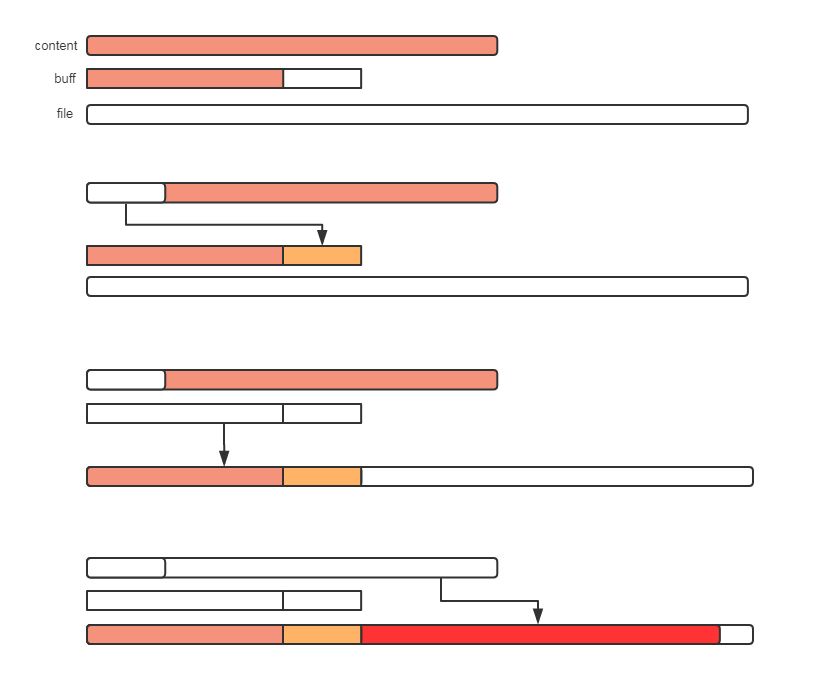### Go语言

Go是一种新的语言，一种并发的、带垃圾回收的、快速编译的语言。Go是谷歌2009年发布的第二款编程语言。2009年7月份，谷歌曾发布了Simple语言，它是用来开发Android应用的一种BASIC语言。

bufio 包介绍

bufio 是通过缓冲来提高效率

bufio 包实现原理bufio 源码分析

``````
buf     []byte
r, w     int    // buf read and write positions
err     error
lastByte   int
lastRuneSize int
}``````

1. 当缓存区有内容的时，将缓存区内容全部填入p并清空缓存区
2. 当缓存区没有内容的时候且len(p)>len(buf),即要读取的内容比缓存区还要大，直接去文件读取即可
3. 当缓存区没有内容的时候且len(p)<len(buf),即要读取的内容比缓存区小，缓存区从文件读取内容充满缓存区，并将p填满（此时缓存区有剩余内容）
4. 以后再次读取时缓存区有内容，将缓存区内容全部填入p并清空缓存区（此时和情况1一样）

``````
// It returns the number of bytes read into p.
// The bytes are taken from at most one Read on the underlying Reader,
// hence n may be less than len(p).
// At EOF, the count will be zero and err will be io.EOF.
n = len(p)
if n == 0 {
}
if b.r == b.w {
if b.err != nil {
}
if len(p) >= len(b.buf) {
// Read directly into p to avoid copy.
if n < 0 {
}
if n > 0 {
b.lastByte = int(p[n-1])
b.lastRuneSize = -1
}
}
// Do not use b.fill, which will loop.
b.r = 0
b.w = 0
if n < 0 {
}
if n == 0 {
}
b.w += n
}

// copy as much as we can
n = copy(p, b.buf[b.r:b.w])
b.r += n
b.lastByte = int(b.buf[b.r-1])
b.lastRuneSize = -1
return n, nil
}

``````

Writer对象

bufio.Writer 是bufio中对io.Writer 的封装

``````
// Writer implements buffering for an io.Writer object.
type Writer struct {
err error
buf []byte
n  int
wr io.Writer
}
``````

bufio.Write(p []byte) 的思路如下

1. 判断buf中可用容量是否可以放下 p
2. 如果能放下，直接把p拼接到buf后面，即把内容放到缓冲区
3. 如果缓冲区的可用容量不足以放下，且此时缓冲区是空的，直接把p写入文件即可
4. 如果缓冲区的可用容量不足以放下，且此时缓冲区有内容，则用p把缓冲区填满，把缓冲区所有内容写入文件，并清空缓冲区
5. 判断p的剩余内容大小能否放到缓冲区，如果能放下（此时和步骤1情况一样）则把内容放到缓冲区
6. 如果p的剩余内容依旧大于缓冲区，（注意此时缓冲区是空的，情况和步骤2一样）则把p的剩余内容直接写入文件

``````
// Write writes the contents of p into the buffer.
// It returns the number of bytes written.
// If nn < len(p), it also returns an error explaining
// why the write is short.
func (b *Writer) Write(p []byte) (nn int, err error) {
for len(p) > b.Available() && b.err == nil {
var n int
if b.Buffered() == 0 {
// Large write, empty buffer.
// Write directly from p to avoid copy.
n, b.err = b.wr.Write(p)
} else {
n = copy(b.buf[b.n:], p)
b.n += n
b.flush()
}
nn += n
p = p[n:]
}
if b.err != nil {
return nn, b.err
}
n := copy(b.buf[b.n:], p)
b.n += n
nn += n
return nn, nil
}
``````

b.wr 存储的是一个io.writer对象，实现了Write()的接口，所以可以使用b.wr.Write(p) 将p的内容写入文件

b.flush() 会将缓存区内容写入文件，当所有写入完成后，因为缓存区会存储内容，所以需要手动flush()到文件

b.Available() 为buf可用容量，等于len(buf) - nGolang中Set类型的实现方法示例详解

go语言中sort包的实现方法与应用详解

Golang中channel使用的一些小技巧
Go语言中接口组合的实现方法
golang中单向channel的语法介绍
golang中interface接口的深度解析
Golang中数据结构Queue的实现方法详解
Golang中List的实现方法示例详解